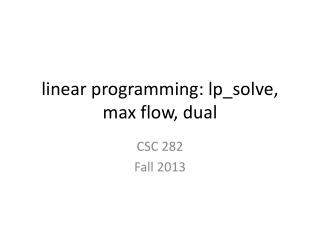DownloadDownload Presentationlinear programming: lp_solve , max flow, dual

# linear programming: lp_solve , max flow, dual

Télécharger la présentation## linear programming: lp_solve , max flow, dual

- - - - - - - - - - - - - - - - - - - - - - - - - - - E N D - - - - - - - - - - - - - - - - - - - - - - - - - - -
##### Presentation Transcript

1. linear programming: lp_solve, max flow, dual CSC 282 Fall 2013

2. lp_solve • lp_solve is a free linear (integer) programming solver based on the revised simplex method and the Branch-and-bound method for the integers • http://lpsolve.sourceforge.net/

3. Example: Profit Maximization

4. run lp_solve

5. run lp_solve

6. lp_solve • free variable – may be negative free x1, x2 ; • converting ratios to LP: (a1 + a2) / (b1 + b2) <= 10 where b1 and b2 are positive becomes (a1 + a2) <= 10 (b1 + b2) • integer and binary variables int r1; bin b1;

7. Flows in Networks Flow Constraints Maximizing Flow

8. Certificate of Optimality • A solution returned by simplex can be converted into a short proof of optimality • s-t cut: disjoint partitioning of vertices into sets L and R • capacity of a cut: total capacity of edges from L to R • optimal flow size(f) ≤ capacity(L,R) for any s-t cut • max-flow min-cut theorem: size of the max flow equals capacity of smallest s-t cut

9. Duality • In flow networks • flows are smaller than cuts, but • max flow = min cut • This is a general property of LP • Every LP max problem has a dual min problem • The solution to the min problem is a proof of optimality of the max problem, and vice-versa

10. Example • (x1,x2) = (100, 300) with objective value 1900 is solution found by simplex to: • Multiplying the three inequalities by 0, 5, 1 respectively and adding them up gives: